用户名 Email 自动登录 找回密码 密码 立即注册## 顺序栈

```class SqStack
{
public \$data;
public \$top;
}```

```function InitSqStack()
{
\$stack = new SqStack();
\$stack->data = [];
\$stack->top = -1;
return \$stack;
}```

```function PushSqStack(SqStack &\$stack, \$x){
\$stack->top ++;
\$stack->data[\$stack->top] = \$x;
}

function PopSqStack(SqStack &\$stack){
// 栈空
if(\$stack->top == -1){
return false;
}

\$v = \$stack->data[\$stack->top];
\$stack->top--;
return \$v;
}```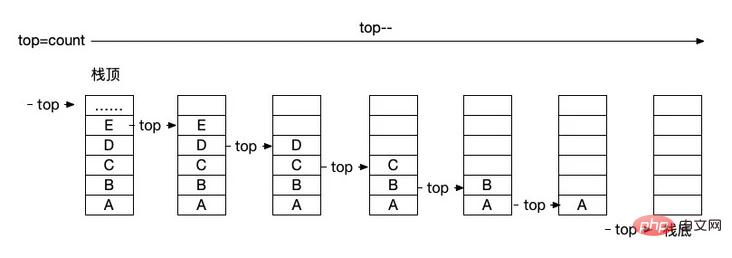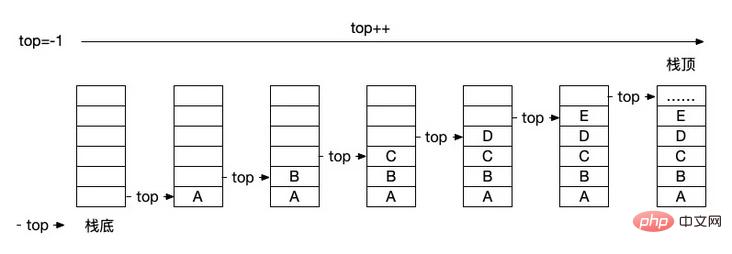```\$stack = InitSqStack();

PushSqStack(\$stack, 'a');
PushSqStack(\$stack, 'b');
PushSqStack(\$stack, 'c');

var_dump(\$stack);
// object(SqStack)#1 (2) {
//     ["data"]=>
//     array(3) {
//       =>
//       string(1) "a"
//       =>
//       string(1) "b"
//       =>
//       string(1) "c"
//     }
//     ["top"]=>
//     int(2)
//   }

echo PopSqStack(\$stack), PHP_EOL; // c
echo PopSqStack(\$stack), PHP_EOL; // b
echo PopSqStack(\$stack), PHP_EOL; // a

var_dump(\$stack);
// object(SqStack)#1 (2) {
//     ["data"]=>
//     array(3) {
//       =>
//       string(1) "a"
//       =>
//       string(1) "b"
//       =>
//       string(1) "c"
//     }
//     ["top"]=>
//     int(-1)
//   }```

## 链栈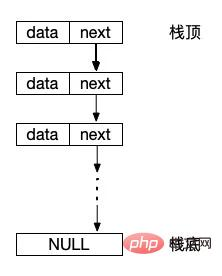```class LinkStack{
public \$data;
public \$next;
}```

```function InitLinkStack(){
return null;
}

\$s->data = \$x;
\$s->next = \$stack;
\$stack = \$s;
}

if(\$stack == NULL){
return false;
}
\$v = \$stack->data;
\$stack = \$stack->next;
return \$v;
}```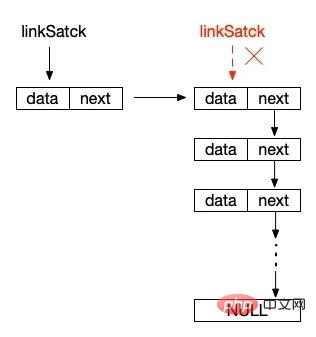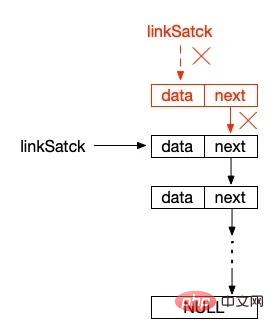```\$stack = InitLinkStack();

var_dump(\$stack);
//     ["data"]=>
//     string(1) "c"
//     ["next"]=>
//       ["data"]=>
//       string(1) "b"
//       ["next"]=>
//         ["data"]=>
//         string(1) "a"
//         ["next"]=>
//         NULL
//       }
//     }
//   }

var_dump(\$stack);
// NULL```

## PHP 为我们提供的数组栈操作

```\$sqStackList = [];

array_push(\$sqStackList, 'a');
array_push(\$sqStackList, 'b');
array_push(\$sqStackList, 'c');

print_r(\$sqStackList);
// Array
// (
//      => a
//      => b
//      => c
// )

array_pop(\$sqStackList);
print_r(\$sqStackList);
// Array
// (
//      => a
//      => b
// )

echo count(\$sqStackList) > 0 ? \$sqStackList[count(\$sqStackList) - 1] : false, PHP_EOL;
// b

array_pop(\$sqStackList);

echo count(\$sqStackList) > 0 ? \$sqStackList[count(\$sqStackList) - 1] : false, PHP_EOL;
// c

array_pop(\$sqStackList);

print_r(\$sqStackList);
// Array
// (
// )```

## 总结

`https://github.com/zhangyue0503/Data-structure-and-algorithm/blob/master/3.栈和队列/source/3.1栈的相关逻辑操作.php`

推荐文章
• 热门
• 最新